International Journal of Energy and Power Engineering
Volume 5, Issue 1-1, February 2016, Pages: 37-41

Fixed-Point Harmonic-Balanced Method for Nonlinear Eddy Current Problems

Xiaojun Zhao1, Yuting Zhong1, Dawei Guan1, Fanhui Meng1, Zhiguang Cheng2

1Department of Electrical Engineering, North China Electric Power University, Baoding, China

2Institute of Power Transmission and Transformation Technology, Baobian Electric Co., Ltd, Baoding, China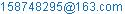(Xiaojun Zhao)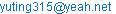(Yuting Zhong)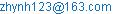(Dawei Guan)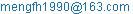(Fanhui Meng)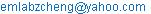(Zhiguang Cheng)

Xiaojun Zhao, Yuting Zhong, Dawei Guan, Fanhui Meng, Zhiguang Cheng. Fixed-Point Harmonic-Balanced Method for Nonlinear Eddy Current Problems. International Journal of Energy and Power Engineering. Special Issue: Numerical Analysis, Material Modeling and Validation for Magnetic Losses in Electromagnetic Devices. Vol. 1, No. 1, 2016, pp. 37-41. doi: 10.11648/j.ijepe.s.2016050101.15

Abstract: A new method to optimally determine the fixed-point reluctivity is presented to ensure the stable and fast convergence of harmonic solutions. Nonlinear system matrix is linearized by using the fixed-point technique, and harmonic solutions can be decoupled by the diagonal reluctivity matrix. The 1-D and 2-D non-linear eddy current problems under DC-biased magnetization are computed by the proposed method. The computational performance of the new algorithm proves the validity and efficiency of the new algorithm. The corresponding decomposed method is proposed to solve the nonlinear differential equation, in which harmonic solutions of magnetic field and exciting current are decoupled in harmonic domain.

Keywords: Eddy Current, Fixed-Point, Harmonic solutions, Reluctivity

1. Introduction

Non-linear eddy current problems can be solved by the time-stepping method  or the harmonic-balanced method . The time-stepping method requires many periods to approach the accurate steady-state solution, while the harmonic-balanced method computes the magnetic field directly in the frequency domain. Compared with the frequency-domain method, the so called brute force method using time-stepping technique spends much more time on the transient process.

A relationship between magnetic field intensity H and the magnetic induction B is represented by introducing the fixed-point reluctivity νFP. The fixed-point reluctivity νFP can be regarded as a periodic variable or a constant in harmonic-balanced method. The convergent speed of the solutions depends mainly on the strategies to determine the value of νFP in non-linear iterations. Different methods to optimally determine νFP have been presented and investigated in order to ensure the stable and fast convergence of solutions [4-6].

When the power transformer works under DC-biased magnetization, the ferromagnetic core will be significantly saturated. Owing to the nonlinearity of magnetic material in electromagnetic devices, there often are high-order harmonics in the exciting current and magnetic field. Therefore, electrical devices such as power transformers and reactors may work abnormally due to the magnetic storm and the transmission of high voltage direct current . In that case, the DC-biased eddy current problem should be solved efficiently and accurately. Furthermore, electromagnetic coupling should always be considered in numerical computation when the strand coils or solid conductors are connected to the voltage source.

In this paper an efficient algorithm to determine the fixed-point reluctivity νFP is proposed. It is aimed at efficiently computing the non-linear eddy current problem under DC-biased magnetization. The nonlinear magnetic field is computed in harmonic domain. The corresponding decomposed algorithm is presented to solve the nonlinear differential equation sequentially or concurrently, which decreases the memory cost of harmonic-balanced computation of large scale problems.

2. Fixed-Point Harmonic-Balanced Method

2.1. Fixed-Point Method

Maxwell’s equations hold in non-linear eddy current problems,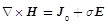(1)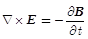(2)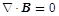(3)

where E is the electric field intensity, J0 is the impressed current density and σ is the conductivity.

A relationship between magnetic field intensity H and magnetic flux density B is represented by introducing the fixed-point reluctivity νFP ,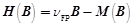(4)

where M is a magnetization-like quantity which varies nonlinearly with B. Therefore the magnetic field intensity H is split into two parts: the linear part, which is related to the νFP, and the non-linear part, which varies with the magnetic induction B .

2.2. Harmonic-Balanced Method

The periodic variables in the electromagnetic field under DC-biased excitation can be approximated by the Fourier-series with a finite number of harmonics ,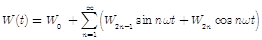(5)

where W(t) can be replaced by current density J, vector potential A, magnetic flux density B, magnetic field intensity H, and the magnetization-like quantity M.

Equation (1) can be rewritten in isotropic material by means of harmonic vector in 2-D problems,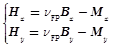(6)

in which(7)

Each of harmonic vectors Bx, By, Mx and My has a similar expression with (7).

2.3. 2-D Eddy Current Problems

Non-linear eddy current problems can be solved directly with the prescribed impressed current density. However, the impressed current density is unknown when the solid conductor or strand coil is connected to the voltage source. Therefore, the coupling between the magnetic field and electric circuits should be investigated if the non-linear eddy current problem is solved in the harmonic domain.

2.3.1. Solid Conductor Connected to Voltage Sources

When the solid conductor is fed by the voltage source, the eddy current exists in the solid conductor and the other conducting materials. The non-linear problem can be described by the following equation,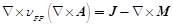(8)

where J is the current density.

The magnetic vector potential A and the scalar potential V can be linked to the current density J by the equation as follows,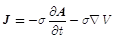(9)

The non-linear equation, including the 2-D magnetic and electric fields, can be presented as follows ,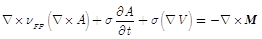(10)

The fixed-point harmonic-balanced equation can be established by applying the finite element method on the whole problem domain,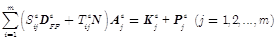(11)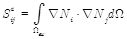(12)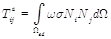(13)(14)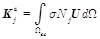(15)

where Ωew, Ωed and Ωen represent the finite element in the whole problem domain, eddy current region and the non-linear region, respectively. Ni is the shape function on node i in each finite element, and m is the total number of nodes in one element. DFP and N are the square matrices related to the fixed-point reluctivity and harmonic number , respectively. Aj is the harmonic vector of the magnetic vector potential on node j and Pj is the harmonic vector obtained from M. U is the voltage in harmonic domain per unit length.

By integrating (13) on the solid conductor, we can obtain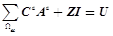(16)

since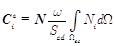(17)(18)

where Ωec represents the finite element in the conducting region, R is the conductor’s resistance per unit length, Scd is the cross-sectional area of the solid conductor.

2.3.2. Strand Coil Connected to Voltage Sources

The strand coil consists of fine wires where the eddy current is generally too small to be considered for computation. The supplied voltage U and the exciting current I in the coil can be linked by Kirchhoff’s Law and Faraday’s Law ,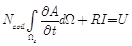(19)

where Ncoil is the turn number of the strand coil.

The frequency-domain system equation considering electromagnetic coupling can be obtained according to the harmonic-balanced theory,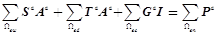(20)(21)

since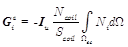(22)

where Iu is a unit matrix of the same size with DFP and N.

Consequently, the harmonic solutions of the magnetic field and magnetizing current can be computed simultaneously by solving (21) when the solid conductor and strand coil are both connected to the voltage source,(23)

where G is related to the spatial distribution of the magnetizing current when the strand coil is fed by the voltage source, while K appears on the right side of the equation when the solid conductor is connected to the voltage source.

2.4. Determination of Fixed-Point Reluctivity

The fixed-point reluctivity νFP can be regarded as a periodic variable when it is determined in each time step. Consequently, the harmonic coefficients of νFP can be used to calculate DFP in the harmonic-balanced method . All elements in the square matrix DFP are non-zero, which indicates harmonic solutions are coupled with each other. In that case the memory demand will increase significantly in the large-scale computation, although fast convergence is achieved. In fact the νFP can be a constant in the harmonic domain, and is determined as follows,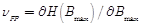(24)

where Bmax represents the maximum value of the magnetic induction in one period.

The mean (emean) and maximum (emax) variation of the reluctivity defined by ν=H/B can be observed to check the convergence of the harmonic solutions. In this paper the stopping criterions are set to emean = 0.1% and emax = 1% in the numerical computation of the one-dimensional and two-dimensional eddy current problems.

3. Computational Results and Analysis

3.1. Laminated Steel Sheet

As shown in Fig.1, A thin electrical steel sheet carrying eddy current is modelled and computed to observe variation of the magnetic induction when the lamination operates under different types of magnetization.

The 30Q140 oriented steel sheet of 0.3 mm thickness is first tested under sinusoidal flux in 50 Hz, and then the DC flux is provided. The conductivity of the sheet is σ = 2.22×106S/m.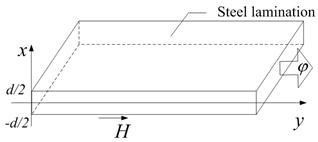Figure 1. Electrical steel sheet.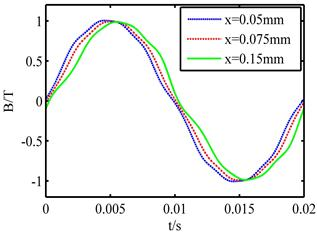Figure 2. Magnetic induction in different depths of the steel sheet.

The spatial distribution of magnetic induction in the thin sheet is well clarified in Fig. 2. The magnetic flux for the boundary condition is Bav,ac = 0.994T and Bav,dc = 0.7036T. Fig. 3 compares the waveforms of magnetic induction under sinusoidal (indicated by "ac") and dc-biased (indicated by "dc") magnetizations. Notice that the eddy current in the sheet leads to the non-sinusoidal waveform of the magnetic induction. Furthermore, the distribution of the magnetic induction varies with the depth of the sheet in the x-direction.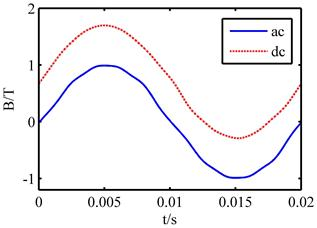Figure 3. Comparison of the magnetic induction under sinusoidal and dc-biased magnetizations.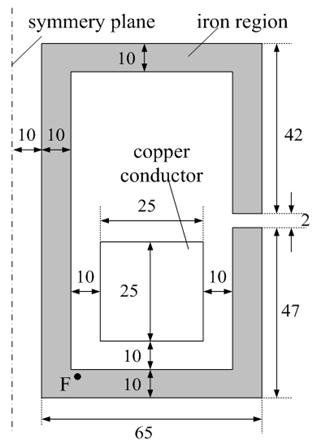Figure 4. Geometric structure of the 2-D model.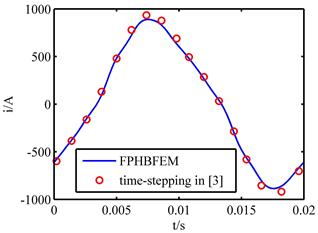Figure 5. Calculated current in the copper conductor.

3.2. Copper Conductor Surrounded by Ferromagnetic Screen

The two-dimensional problem consists of a solid copper conductor and an iron screen with an air gap. As shown in Fig. 4, the iron screen surrounds the conductor. The eddy current exists in both the copper conductor and iron screen. The conductivities of the copper and iron are σ= 5.7×107S/m and σ = 1.0×106S/m, respectively. The copper conductor is connected to a voltage source of 50 Hz. The B-H curve is detailed in . 892 second-order elements with 2781 nodes are used in the numerical computation. Computational costs of the proposed method and the traditional method  are compared in Table I. Mc and Tc represent the memory demand and computational time, respectively. Nh is the truncated harmonic number. Compared to the traditional method, the proposed method significantly reduces memory requirements with a slight increase in computational time due to a few more non-linear iterations. The calculated magnetizing current is compared with that obtained by using time-stepping method, and the good congruency proves the validity of the proposed method.

Table 1. Comparison between two different methods.

 Method Traditional Proposed Memory/Mb 17.51 7.92 Time/s 951.31 812.86 Nh 11 11

4. Decomposed Algorithm

When the fixed-point reluctivity is computed according to (24), the nonlinear equation in (23) can be linearized by νFP which is space-dependent and time-independent. Therefore harmonic solutions can be decoupled and calculated separately. Equation (11) can be decomposed as follows,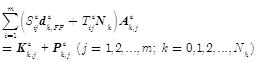(25)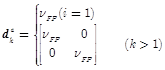(26)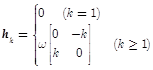(27)

where k is the harmonic number.

The decoupled equation system can be solved sequentially and concurrently, updating harmonic solutions by Gauss-Seidel and Jacobi iterative method respectively.

5. Conclusion

The convergent speed of harmonic solutions highly depends on the determination of optimal fixed point reluctivity in the fixed-point harmonic-balanced method. Differential reluctivity can be used to guarantee the stable and fast convergence of harmonic solutions. Due to the linearized system equation, harmonic solutions can be decoupled and computed in parallel, which can improve the computational efficiency and reduce the memory cost greatly. The proposed algorithm is more efficient than the traditional harmonic-balanced method.

Acknowledgement

This work is supported by the National Natural Science Foundation of China (Grant No. 51307057), Hebei Province Natural Science Foundation (Grant No.E2013502323), Research Fund for the Doctoral Program of Higher Education of China (Grant No. 20130036120011), and the Fundamental Research Funds for the Central Universities (Grant No. 2015MS82).

References

1. E. Dlala, A. Belahcen, and A. Arkkio, "Locally convergent fixed-point method for solving time-stepping nonlinear field problems," IEEE Trans. Magn., vol.43, pp. 3969-3975, 2007.
2. X. Zhao, L. Li, J. Lu, Z. Cheng and T. Lu, "Characteristic analysis of the square laminated core under dc-biased magnetization by the fix-point harmonic-balanced mehtod," IEEE Trans. Magn., vol. 48, no. 2, pp. 747-750, 2012.
3. O. Biro and K. Preis, "An efficient time domain method for nonlinear periodic eddy current problems," IEEE Trans. Magn., vol. 42, no. 4, pp. 695-698, 2006.
4. E. Dlala and A. Arkkio, "Analysis of the convergence of the fixed-point method used for solving nonlinear rotational magnetic field problems," IEEE Trans. Magn., vol. 44, no. 4, pp. 473-478, 2008.
5. S. Ausserhofer, O. Biro, and K. Preis, "A strategy to improve the convergence of the fixed-point method for nonlinear eddy current problmes," IEEE Trans. Magn., vol. 44, no. 6, pp. 1282-1285, 2008.
6. G. Koczka, S. Auberhofer, O. Biro and K. Preis, "Optimal convergence of the fixed point method for nonlinear eddy current problmes," IEEE Trans. Magn., vol. 45, no. 3, pp. 948-951, 2009.
7. X. Zhao, J. Lu, L. Li, Z. Cheng and T. Lu, "Analysis of the saturated electromagnetic devices under DC bias condition by the decomposed harmonic balance finite element method", COMPEL., vol. 31, no. 2, pp. 498-513, 2012.
8. F. I. Hantila, G. Preda and M. Vasiliu, "Polarization method for static field" IEEE Trans. Magn., vol.36, no.4, pp. 672-675, 2000.
9. X. Zhao, J. Lu, L. Li, Z. Cheng and T. Lu, "Analysis of the DC Bias phenomenon by the harmonic balance finite-element method," IEEE Trans. on Power Delivery., vol.26, no.1, pp. 475-485, 2011.
10. I. Ciric, and F. Hantila, "An efficient harmonic method for solving nonlinear time-periodic eddy-current problmes," IEEE Trans. Magn., vol. 43, no. 4, pp. 1185-1188, 2007.
11. P. Zhou, W. N. Fu, D. Lin, and Z. J. Cendes, "Numerical modeling of magnetic devices," IEEE Trans. Magn., vol. 40, no. 4, pp. 1803–1809, 2004.

 Contents 1. 2. 2.1. 2.2. 2.3. 2.4. 3. 3.1. 3.2. 4. 5.
Article ToolsAbstractPDF(789K)